# Deep Learning: Predicting the average income of residents in California.

in hive-174578 •  27 days ago

We can train the computer to predict the average income of people by considering a combination of different factors such as their residential neighbourhood, house size, house age, population of the city/town. I have written a code which can predict with about 98% accuracy the average income of residents of California.

Let's discuss the code here.

``````import torch
import jovian
import torchvision
import torch.nn as nn
import pandas as pd
import numpy as np
import matplotlib.pyplot as plt
import torch.nn.functional as F
from torch.utils.data import DataLoader, TensorDataset, random_split

``````

Here I am importing required PyTorch modules.

``````batch_size=64
learning_rate=1e-5

# Other constants
DATASET_URL = "/kaggle/input/housing.csv"
DATA_FILENAME = "housing.csv"
TARGET_COLUMN = 'ocean_proximity'
input_size=9
output_size=1

``````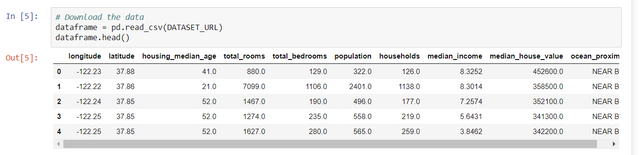First we need to gather as much data as possible about the residents of California. The dataset can be in .csv file.

``````def customize_dataset(dataframe_raw):
dataframe = dataframe_raw.copy(deep=True)
# drop some columns
dataframe = dataframe.drop(['longitude', 'latitude'], axis=1)
#for col in ['housing_median_age', 'total_rooms', 'total_bedrooms', 'population',
#            'households', 'median_income' ,'median_house_value']:
#    # normalizing incoming data
#    dataframe[col] = (dataframe[col] - min(dataframe[col])) / (max(dataframe[col]) - min(dataframe[col]))

# dropping any row that contains at least on missing value
# if you dont do that, loss function will be returning nan
dataframe = dataframe.dropna(axis=0)

return dataframe

``````

Before we begin training the model, we need to look at the data and sometimes we may need to customise the data to increase the accuracy of the result. In this case, I have excluded partially missing information in the dataset which has helped predict the accuracy.

``````dataframe = customize_dataset(dataframe)

``````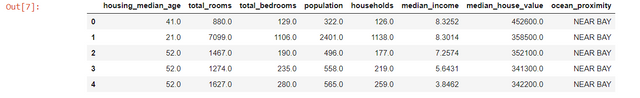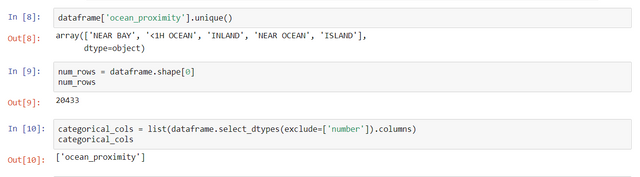``````input_cols = list(dataframe.columns[0:4])+list(dataframe.columns[-1:])

input_cols

``````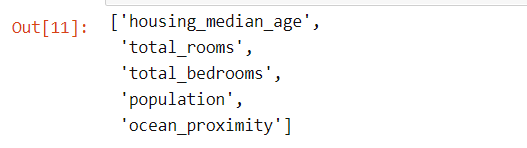``````output_cols = list(dataframe.columns[5:6])
print(len(output_cols))
print(output_cols)

``````After customising the data, we are ready to begin coding in the Jupyter notebook. Then, we segregate the data on which we will train our model.

``````# Convert from Pandas dataframe to numpy arrays

def dataframe_to_arrays(dataframe):
# Make a copy of the original dataframe
dataframe1 = dataframe.copy(deep=True)
# Convert non-numeric categorical columns to numbers
for col in categorical_cols:
dataframe1[col] = dataframe1[col].astype('category').cat.codes
# Extract input & outupts as numpy arrays
inputs_array = dataframe1[input_cols].to_numpy()
targets_array = dataframe1[output_cols].to_numpy()
return inputs_array, targets_array

inputs_array, targets_array = dataframe_to_arrays(dataframe)
inputs_array, targets_array
``````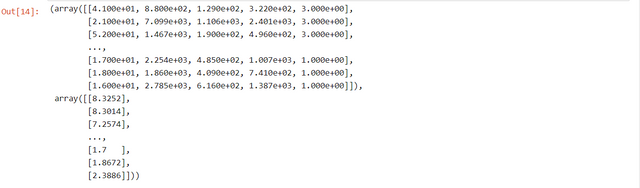We now convert the segregated data to Numpy arrays.

``````#To convert Numpy arrays to PyTorch Tensors

inputs = torch.from_numpy(inputs_array).type(torch.float32)
targets = torch.from_numpy(targets_array).type(torch.float32)

``````

We will be using PyTorch to train our model so we need to convert the NumPy arrays into PyTorch tensors.

``````inputs.dtype, targets.dtype
dataset = TensorDataset(inputs, targets)
val_percent = 0.1 # between 0.1 and 0.2
val_size = int(num_rows * val_percent)
train_size = num_rows - val_size

train_ds, val_ds = random_split(dataset, [train_size, val_size]) # Use the random_split function to split dataset into 2 parts of the desired length

print("inputs:", xb)
print("targets:", yb)
break
``````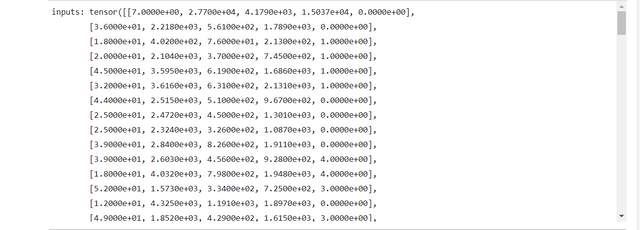``````input_size = len(input_cols)
output_size = len(output_cols)
print(len(input_cols))
print(len(output_cols))

``````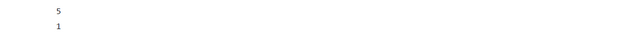We can train our model with the data which is now in the form of PyTorch tensors.

``````class HousingModel(nn.Module):
def __init__(self):
super().__init__()
self.linear = nn.Linear(input_size, output_size)

def forward(self, xb):
out = self.linear(xb)
return out

def training_step(self, batch):
inputs, targets = batch
out = self(inputs)                 # Generate predictions
loss = F.l1_loss(out, targets)    # Calculate loss
return loss

def validation_step(self, batch):
inputs, targets = batch
out = self(inputs)                 # Generate predictions
loss = F.l1_loss(out, targets)    # Calculate loss
return {'val_loss': loss.detach()}

def validation_epoch_end(self, outputs):
batch_losses = [x['val_loss'] for x in outputs]
epoch_loss = torch.stack(batch_losses).mean()   # Combine losses
return {'val_loss': epoch_loss.item()}

def epoch_end(self, epoch, result):
print("Epoch [{}], val_loss: {:.4f}".format(epoch, result['val_loss']))

model = HousingModel()

outputs = [model.validation_step(batch) for batch in val_loader]
return model.validation_epoch_end(outputs)

history = []
optimizer = opt_func(model.parameters(), learning_rate)
for epoch in range(epochs):
# Training Phase
loss = model.training_step(batch)
loss.backward()
optimizer.step()
# Validation phase
model.epoch_end(epoch, result)
history.append(result)
return history

result
``````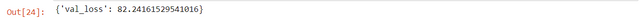``````list(model.parameters())

``````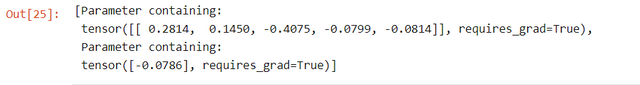Once our model is trained we can make predictions with it.

``````history2= fit(50, 1e-8, model, train_loader, val_loader, opt_func=torch.optim.SGD)
history2

``````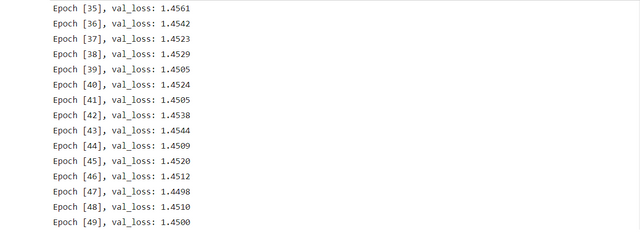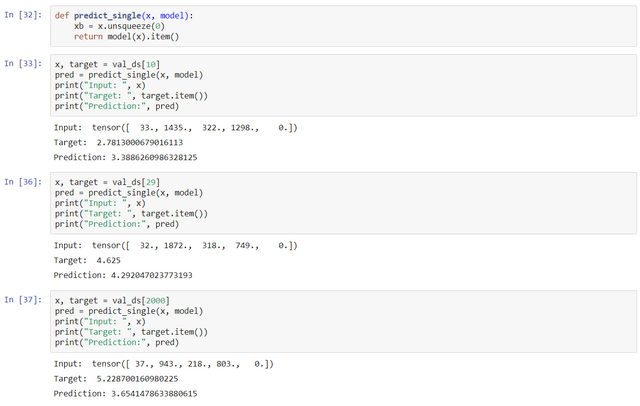To view the Jupyter notebook click here. Feel free to give me any feedback or ask questions.

If you want to get into Deep Learning I highly recommend "Deep Learning with PyTorch: Zero to GAN’s” taught by Aakash N S of Jovian.ml.